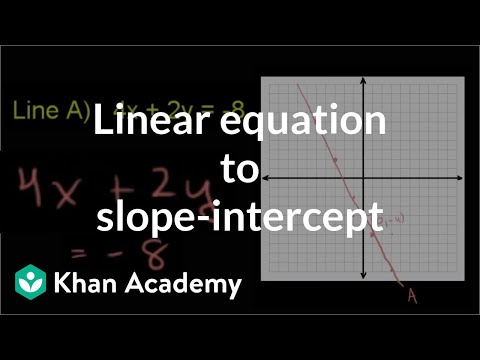# Graphing a linear equation: 5x + 2y = 20 (Full video)

Description: Sal graphs a linear equation by first generating a table of values. Create a graph of the linear equation 5x plus 2y is equal to 20. So the line is essentially the set of all coordinate, all x's and y's, that satisfy this relationship right over here. But to make it a little bit simpler, I'm going to solve for y here.

### Other videos you might be interested in### Ratio and Proportion in Grades 6-8: Connections to College and Career Skills (Full video)

#### The Hunt Institute